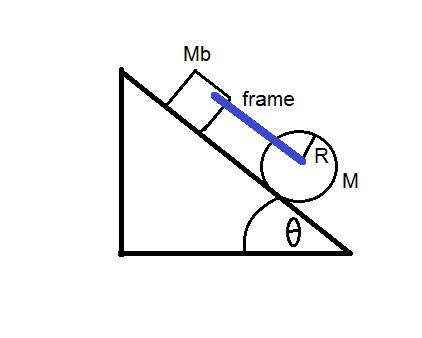# Cylinder attached to a block on an inclined plane, rotational dynamics

• jsrev

## Homework Statement

A cylinder of mass M and radius R, with moment of inertia:
$$I_c = \frac{1}{2}MR^2$$
rolls down without slipping through an inclined plane with an angle of θ, while pulling a block of mass Mb with an attached frame (of insignificant mass) which is connected to the axis of the cylinder.
If the cylinder rolls without slipping over the surface and the coefficient of kinetic friction between the block Mb and the surface is μ, then:

a. Deduct an expression for the linear acceleration of the system.

This problem didn't come with a figure, but I made one based on what I think the system looks like.
See figure http://imgur.com/HyiUSLu

## Homework Equations

Linear acceleration

## The Attempt at a Solution

I've tried a few ways, like calculating the linear acceleration of each object (the cylinder and the block) but there are some things I don't get.
I have tried calculating
$$a_{cylinder} = \frac{gsinθ}{k}$$
given that k is a constant that equal 1/2 from the first equation. Then I got the linear acceleration of the block with $$a_{block} = gsinθ$$ and also considered the friction, but I'm not any close to the answer.

The given solution is:

$$a = \frac{2g[(M+M_b)sinθ-μM_bcosθ]}{3M + 2M_b}$$

If anyone can guide me a little bit in this one I would really appreciate it. Thank you.

## Homework Statement

A cylinder of mass M and radius R, with moment of inertia:
$$I_c = \frac{1}{2}MR^2$$
rolls down without slipping through an inclined plane with an angle of θ, while pulling a block of mass Mb with an attached frame (of insignificant mass) which is connected to the axis of the cylinder.
If the cylinder rolls without slipping over the surface and the coefficient of kinetic friction between the block Mb and the surface is μ, then:

a. Deduct an expression for the linear acceleration of the system.

This problem didn't come with a figure, but I made one based on what I think the system looks like.
See figure http://imgur.com/HyiUSLu

## Homework Equations

Linear acceleration

## The Attempt at a Solution

I've tried a few ways, like calculating the linear acceleration of each object (the cylinder and the block) but there are some things I don't get.
I have tried calculating
$$a_{cylinder} = \frac{gsinθ}{k}$$
given that k is a constant that equal 1/2 from the first equation. Then I got the linear acceleration of the block with $$a_{block} = gsinθ$$ and also considered the friction, but I'm not any close to the answer.

The given solution is:

$$a = \frac{2g[(M+M_b)sinθ-μM_bcosθ]}{3M + 2M_b}$$

If anyone can guide me a little bit in this one I would really appreciate it. Thank you.
Draw a free body diagram for the cylinder, and one for the block.#### Attachments

See post 4
dean barry

Last edited:
Sorry, made a hash of that, here's the revised effort :

Assuming its a homogenous cylinder, then the equivalent mass (Me) of the non slipping cylinder for acceleration calculation purposes = 1.5 * M
(kg)

The total mass (Mt) being accelerated = Me + Mb
(kg)

The force due to gravity driving the system = ( M * g * sine θ ) + ( Mb * g * sine θ )
(Newtons)

The drag force of the block = Mb * g * cosine Ѳ * µ
(Newtons)

So :
The net force (fn) driving the system :
fn = ( ( M * g * sine θ ) + ( Mb * g * sine θ ) ) - ( Mb * g * cosine Ѳ * µ )

The acceleration (a) of the system = fn / Mt
So :
a = ( ( ( M * g * sine θ ) + ( Mb * g * sine θ ) ) - ( Mb * g * cosine Ѳ * µ ) ) / ( Me + Mb )